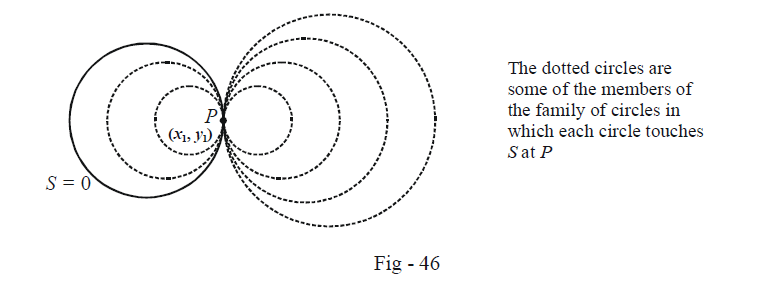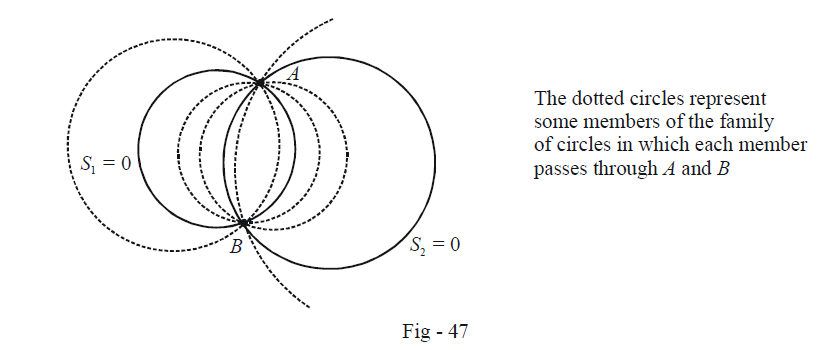# Family Of Circles Type 2 And 3

Go back to  'Circles'

TYPE 2:

\fbox{\begin{align}{{\text{FAMILY OF CIRCLES TOUCHING A GIVEN CIRCLE AT A GIVEN POINT}}}\end{align}}

Let the equation of the fixed circle be

$S:{x^2} + {y^2} + 2gx + 2fy + c = 0$

and let there be a point $$P({x_1},{y_1})$$ lying on this circle. We wish to determine the equation of the family of circles touching S at P.We can write the equation of the tangent to $$S = 0$$ at P as

$T:x{x_1} + y{y_1} + g(x + {x_1}) + f(y + {y_1}) + c = 0$

Once we have a circle $$(S = 0)$$ and a line $$(T = 0)$$ intersecting or touching the circle, we can write the equation of the family of circles passing through the point (s) of intersection of the circle and the line, using the result derived in the last article. Thus, the required family can be represent as

$\fbox{F:S + \lambda T = 0}$

\begin{align}&\Rightarrow \qquad F:{x^2} + {y^2} + 2gx + 2fy + c + \lambda (x{x_1} + y{y_1} + g(x + {x_1}) + f(y + {y_1}) + c = 0\\& \Rightarrow \qquad F:{x^2} + {y^2} + (2g + \lambda {x_1} + \lambda g)x + (2f + \lambda {y_1} + \lambda f)y + c + \lambda g{x_1} + \lambda f{y_1} + \lambda c = 0\end{align}

As we vary $$\lambda ,$$ we will obtain different members belonging to this family.

TYPE 3:

\fbox{\begin{align} & \text{FAMILY OF CIRCLES PASSING THROUGH THE INTERSECTION POINT(S) OF } \\ & \text{TWO GIVEN CIRCLES} \\ \end{align}}

Let the two fixed circles be

\begin{align}&{S_1}:{x^2} + {y^2} + 2{g_1}x + 2{f_1}y + {c_1} = 0\\&{S_2}:{x^2} + {y^2} + 2{g_2}x + 2{f_2}y + {c_2} = 0\end{align}

and their points of intersection be $$A({x_1},{y_1})$$ and $$B({x_2},{y_2}).$$ In case the two circles touch each other, A and B will be the same.

We wish to determine the family of circles passing through A and B.You might be able to extrapolate from the last few cases that the equation representing this family will be

$\fbox{F:{S_1} + \lambda {S_2} = 0}$

You can verify this by writing the equation for F in standard form and observing that it does indeed represent a circle. Also, since (the co-ordinates of) A and B satisfy both $${S_1} = 0$$ and $${S_2} = 0,$$ they have to satisfy the equation for F. Note one important point: $$\lambda$$ cannot be equal to –1 otherwise F will become the common chord of $${S_1} = 0$$ and $${S_2} = 0$$ instead of representing a circle.

Example - 37

Find the equation of the circle which passes through the points of intersection of the circles

\begin{align}&{S_1}:{x^2} + {y^2} - 6x + 2y + 4 = 0\\&{S_2}:{x^2} + {y^2} + 2x - 4y - 6 = 0\end{align}

and whose centre lies on the line y = x.

Solution: Let the required equation be S = 0. Then, by the previous article, we can find some $$\lambda \in \mathbb{R}$$ and $$\lambda \ne - 1$$ such that

\begin{align}& \quad \quad \;\;S \equiv {S_1} + \lambda {S_2} = 0\\ & \Rightarrow \quad S \equiv (1 + \lambda ){x^2} + (1 + \lambda ){y^2} + (2\lambda - 6)x + (2 - 4\lambda )y + 4 - 6\lambda = 0\,\,\,\,\,\,\,\,\,\,\,\,\,\,\,\,\,\,\,\,\,\,\,\,\,\,\,\,\,\,\,\,\,\,\,\,...(1)\end{align}

The centre of S from this equation comes out to be

${\rm{centre}} \equiv \left\{ { - \frac{{(\lambda - 3)}}{{1 + \lambda }},\,\, - \frac{{(1 - 2\lambda )}}{{1 + \lambda }}} \right\}$

Since the centre lies on the line y = x, we have

\begin{align}& - \frac{{(\lambda - 3)}}{{1 + \lambda }} = - \frac{{(1 - 2\lambda )}}{{1 + \lambda }}\\ \Rightarrow \quad & \lambda = \frac{4}{3}\end{align}

We now substitute this value back in (1) to obtain the equation for S:

\begin{align} & S:\frac{7}{3}{x^2} + \frac{7}{3}{y^2} - \frac{{10}}{3}x - \frac{{10}}{3}y - 4 = 0\\ \Rightarrow \quad & S:{x^2} + {y^2} - 10x - 10y - 12 = 0\end{align}